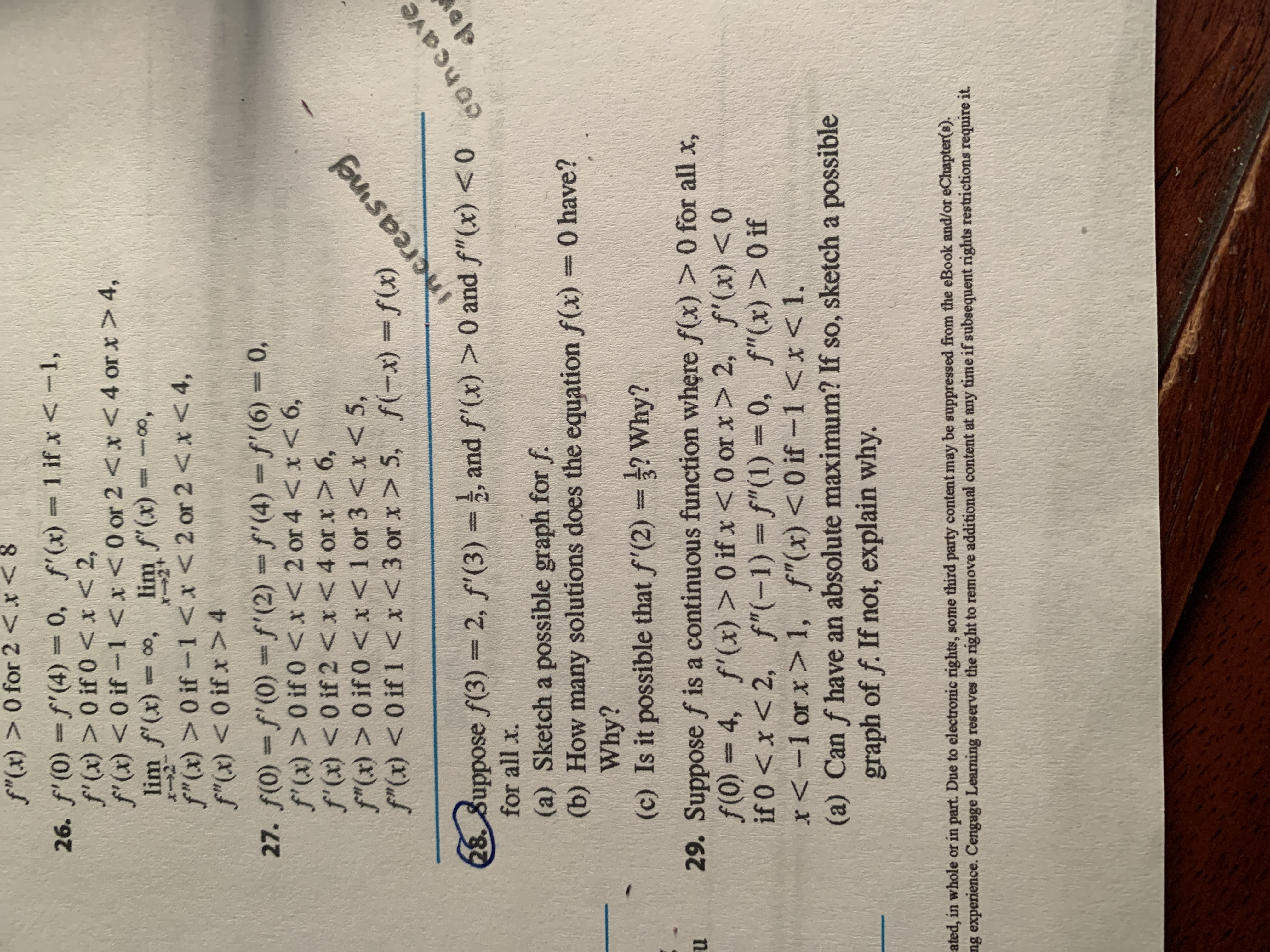# f"(x) > 0 for 2 0 if 0 4,lim f'(x) 00, lim f'(x) -o,f"(x) >0 if-14%3D→2+27. f(0) =f'(0) =f'(2) =f'(4) = f'(6) = 0,f'(x) >0 if 0 6,f"(x) >0 if 0 5, f(-x) f (x)%3Dconcavedow28.Suppose f(3) = 2, f'(3) =, and f'(x) > 0 and f"(x) 0 for all x,f(0) = 4, f'(x) > 0 if x 2, f'(x) 1, f"(x) 0 ifated, in whole or in part. Due to electronic rights, some third party content may be suppressed from the eBook and/or eChapter(s).ng experience. Cengage Leaming reserves the right to remove additional content at any time if subsequent rights restrictions require it.

Question
2 views

Number 28 part Chelp_outlineImage Transcriptionclosef"(x) > 0 for 2 0 if 0 4, lim f'(x) 00, lim f'(x) -o, f"(x) >0 if-14 %3D →2+ 27. f(0) =f'(0) =f'(2) =f'(4) = f'(6) = 0, f'(x) >0 if 0 6, f"(x) >0 if 0 5, f(-x) f (x) %3D concave dow 28.Suppose f(3) = 2, f'(3) =, and f'(x) > 0 and f"(x) <0 increas ng for all x. (a) Sketch a possible graph for f. (b) How many solutions does the equation f(x) 0 have? Why? (c) Is it possible that f'(2) =? Why? 29. Suppose f is a continuous function where f(x) > 0 for all x, f(0) = 4, f'(x) > 0 if x <0 or x> 2, f'(x) <0 if 0 1, f"(x)<0 if -1 0 if ated, in whole or in part. Due to electronic rights, some third party content may be suppressed from the eBook and/or eChapter(s). ng experience. Cengage Leaming reserves the right to remove additional content at any time if subsequent rights restrictions require it. fullscreen
check_circle

Step 1

Given that, f " (x)<0 for all x.

This me...

### Want to see the full answer?

See Solution

#### Want to see this answer and more?

Solutions are written by subject experts who are available 24/7. Questions are typically answered within 1 hour.*

See Solution
*Response times may vary by subject and question.
Tagged in